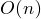## Bucket Sort Back

• 桶排序: 把所有數分成若干區間, 並為每個區間建立鏈表. 把所有數分別加入對應區間, 並對所有鏈進行穩定排序
• 时间复杂度:(最好,平均,最壞情況)
• 空間複雜度: /
• 稳定性: 稳定
• 适用情况: 數的分佈均勻
• 散列過程:``````/* [0,1) */
void BUCKETSORT(int A[], int array_size)
{
int n = array_size;
for (int i = 1; i <= n; i++)
//insert_list(B[n*A[i]], A[i]);
for (int i = 0; i <= n - 1; i++)
INSERTIONSORT(B[i], B[i].array_size);
}
``````